### 8.4.4.1 An acceleration-based control model

To motivate the introduction of constraints, consider a control model proposed in [235,830]. The action space, defined as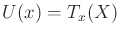in Formulation 8.2, produces a velocity for each action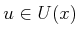. Therefore,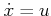. Suppose instead that each action produces an acceleration. This can be expressed as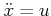, in which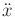is an acceleration vector,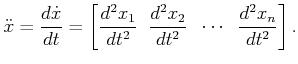(8.44)

The velocity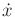is obtained by integration over time. The state trajectory,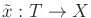, is obtained by integrating (8.44) twice.

Suppose that a vector field is given in the form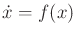. How can a feedback plan be derived? Consider how the velocity vectors specified by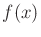change asvaries. Assume thatis smooth (or at least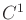), and let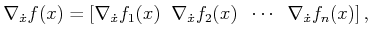(8.45)

in which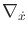denotes the unnormalized directional derivative in the direction of: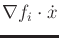. Suppose that an initial state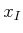is given, and that the initial velocity is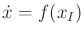. The feedback plan can now be defined as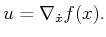(8.46)

This is equivalent to the previous definition of a feedback plan from Section 8.4.1; the only difference is that now two integrations are needed (which requires bothandas initial conditions) and a differentiability condition must be satisfied for the vector field.

Now the relationship betweenandwill be redefined. Suppose thatis the true measured velocity during execution and thatis the prescribed velocity, obtained from the vector field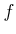. During execution, it is assumed thatandare not necessarily the same, but the task is to keep them as close to each other as possible. A discrepancy between them may occur due to dynamics that have not been modeled. For example, if the fieldrequests that the velocity must suddenly change, a mobile robot may not be able to make a sharp turn due to its momentum.

Using the new interpretation, the difference,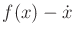, can be considered as a discrepancy or error. Suppose that a vector fieldhas been computed. A feedback plan becomes the acceleration-based control model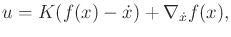(8.47)

in which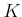is a scalar gain constant. A larger value ofwill make the control system more aggressively attempt to reduce the error. Ifis too large, then acceleration or energy constraints may be violated. Note that if, then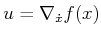, which becomes equivalent to the earlier formulation.

Steven M LaValle 2020-08-14